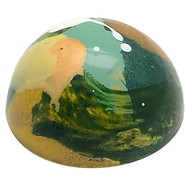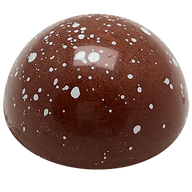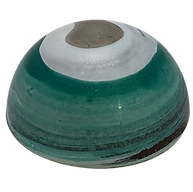top of page

#### Chocolates

Currenty out of stockC a r a m e l  &  L i m eR a s p b e r r y  &  C o c o n u tC o f f e eM a t c h a  T e aC o o k i e s  &  C r e a mM a p l e  &  P e c a nA p r i c o t  &  Y o g u r tP a s s i o n  F r u i tF r e s h  M i n tR o a s t e d  H a z e l n u tP e a n u t  B u t t e r  &  J a mS a l t e d  C a r a m e l

The chocolate bonbons are hand-made in house with high-quality chocolate. Our flavours can change depending on our moods.

Contact us if you want to know what we have in stock.

bottom of page